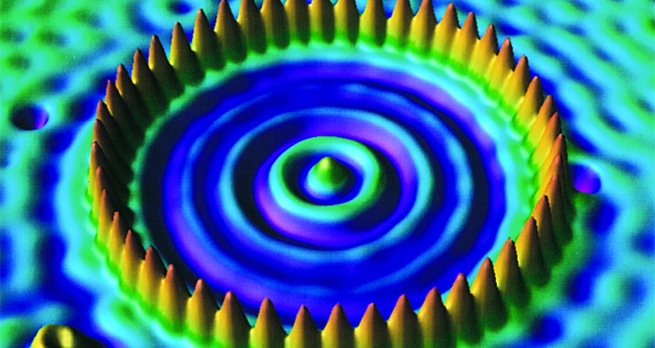Collisions and conservation laws

Start this free course now. Just create an account and sign in. Enrol and complete the course for a free statement of participation or digital badge if available.

Free course

# 5 Inelastic collisions

We now extend our discussion to include inelastic cases, where the total kinetic energy changes during the one-dimensional collision.

First we consider the case where the two particles stick together on impact; this is an example of a completely inelastic collision, which occurs with the maximum loss of kinetic energy consistent with conservation of momentum. As a simple example, suppose we have two bodies of equal mass, with one initially at rest. If the initial velocity of the other is and the initial momentum is , the final momentum must be the same so, since the mass has been doubled, the final velocity is and the final kinetic energy is therefore

Since the initial kinetic energy was twice as large as this, it follows that half the original kinetic energy has been lost (mainly as thermal energy), during the collision.

For the more general case where the colliding masses are unequal, but they stick together at collision, we still have and so momentum conservation implies that

and provides a full solution of the problem (a value for ), without recourse to energy.

To complete the picture, let us mention the general case where two particles collide but where the transfer of kinetic energy into other forms is less than that for the completely inelastic case. This problem has no general solution without more information, such as the fraction of kinetic energy converted. Such problems have solutions which lie between those for the two extremes of elastic and completely inelastic collisions but they must be tackled on an individual basis, using the general principles of conservation of momentum and energy.

You will see that in all these calculations we have not needed to invoke the rather complicated forces involved in the interaction of the two particles, but rather have been able to solve the problems using only the principles of conservation of momentum and energy. This is a great simplification and illustrates the power of using conservation principles whenever possible.

S217_1